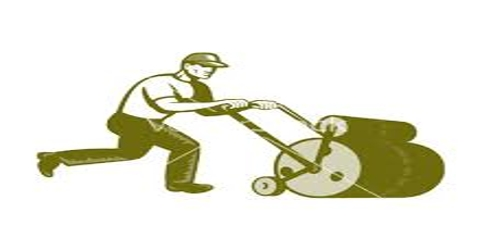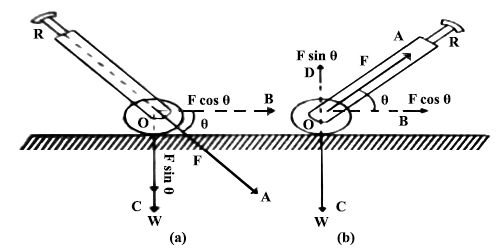# Lawn Roller Example in Vector Addition and Substraction

Lawn Roller: When an object is either pulled or pushed on a plane, a frictional force acts between the plane and the object. This retards the motion of the object. The heavier the object, larger is the frictional force. A lawn roller is made moving either by pushing or pulling.

In case of pushing: Let the weight of the roller be W and the applied force on the handle of the roller be F. Let the force F act at O at angle θ with the horizontal plane [Fig.(a)]. Now, F can be resolved at O into two normal components.

The horizontal component of the force = F Cos θ. which acts along OB in the forward direction and the vertical component of the force – F sin θ, whose direction is along OC. This increases the weight of the roller.

So, the total weight of the roller is. (W + R sin θ), which is larger than the actual weight of the roller. Consequently, the frictional force also increases. So, it is difficult to push the roller.In case of pulling: Let, the weight of the roller = W and the applied force on the handle = F. The force F acts at O at an angle θ along the horizontal line OB

[Fig. (b)]; This force can be resolved into two normal components. The horizontal component of F is F cos θ. Due to its action, the roller moves in the forward direction.

The vertical component of F is F sin θ, which acts upward along OD. So the total weight of the roller decreases. The weight of the roller is (W — F sin θ), which is less than the actual weight of the roller. The frictional force also decreases, so it becomes easier to pull a roller.

Conclusion: It is easier to pull a lawn roller than to push.